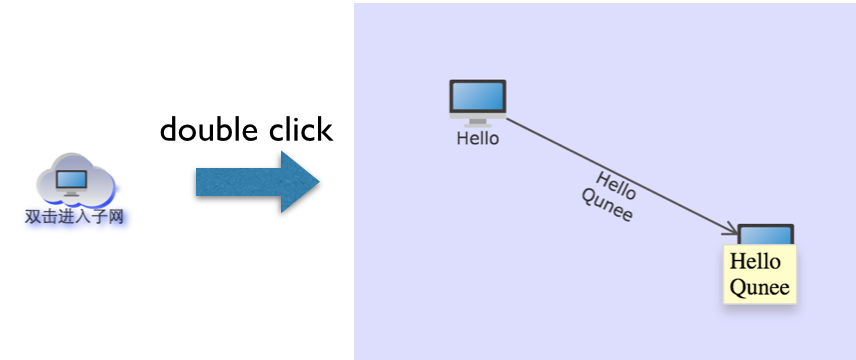Light High Efficiency Graph Component

# Subnet Type

Topological graph can describe the distribution of network structure. One image indicates a network. The network generally has hierarchical relationship. WAN has metropolitan area network. The metropolitan area network has local area network. This kind of affiliate network is the sub network we call (sub network)

In Qunee any element can be regarded as the subnet, such as word, node, edge and even the group. Just hast to set enableSubNetwork property as true. It can be expressed as subnet type.

• Set as the subnet type - any element can be regarded as subnetelement.enableSubNetwork = true;
• enter this subnet
graph.currentSubNetwork = element;
• returns the upper layer of subnet
graph.upSubNetwork();
• monitors the change event of subnet
graph.onPropertyChange(‘currentSubNetwork’, function(evt){ … } )

### Example

``````var graph;
\$(function(){
graph = new Q.Graph('canvas');

var subnetwork = graph.createNode('双击进入子网');
subnetwork.image = Q.Graphs.subnetwork;
subnetwork.enableSubNetwork = true;

var hello = graph.createNode("Hello", -100, -50);
var qunee = graph.createNode("Qunee", 100, 50);
var edge = graph.createEdge("Hello\nQunee", hello, qunee);

hello.parent = subnetwork;
qunee.parent = subnetwork;
edge.parent = subnetwork;

graph.onPropertyChange('currentSubNetwork', function(){
graph.html.style.backgroundColor = graph.currentSubNetwork == subnetwork ? '#DDF' : '#FFF';
});
})
``````

Operation effect